Like   Tweet
 /* styles */ April 29, 2020 Dear Readers, In this fourth installment of “Expand Your Horizons While Sheltering at Home,” we would like to reintroduce you to the 2016 Finalists for the Dayton Literary Peace Prize. Each year, we have noticed a synchronicity connecting the finalists even though the fiction and nonfiction judging are separate. The 2016 books focused on the devastation of war and the power of love, often in the same book. The authors take us to conflicts in Afghanistan, Armenia, Vietnam, Iraq, Africa, Japan, and the United States. They explore powerful stories of the evolution of self-awareness, awakening to truth, love between friends and within families. Through these books we explore our own variant notions of what is acceptable. What code? What religion? What belief? What rules? What ethic? determine our particular behaviors. Just as surely as we can be absorbed into the world of the book we are reading, we simultaneously absorb what we read. We become residents of Gilead and a slum in Nairobi; suffer the empathetic pain of a bomb blast in Nagasaki and having our hands amputated in Louisiana; witness the injustices and racial tyranny in our own country and beyond; a sympathizer reminds us of desperate attempts to make any sense of a war that ravaged Vietnam and shattered the dreams of America. We invite you to meet the characters and people in these powerful books that help us find our own truth. Stay safe,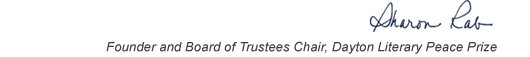table div table+table+table+table div table{width:100%;padding:0}table div table+table+table+table div table img{width:96.23%;padding:0;float:none}table div table+table+table+table div table td{width:100%;padding:0 1.88% 18px}/* styles */This list includes the winners and runners-up for 2016 and we have rich background information on each one on our website.

▪ Under Past Winners. scroll down to the year and click on it.
▪ You will have the option of clicking on a winner, a runner-up, or the finalists.
▪ If you click on the photo of winners and runners-up, you will find the author’s photo and bio, a selection from the book, the judges’ citation, the writer’s reflection on literature and peace, and the option of watching the introduction and acceptance speech.
 ▪ Under Past Winners. scroll down to the year and click on it.
 ▪ You will have the option of clicking on a winner, a runner-up, or the finalists.
 ▪ If you click on the photo of winners and runners-up, you will find the author’s photo and bio, a selection from the book, the judges’ citation, the writer’s reflection on literature and peace, and the option of watching the introduction and acceptance speech.

On the bottom right of the home page, you can click on COREScholar, which is built by the Chair of our Curriculum Committee Carol Loranger, Associate Dean of the College of Liberal Arts at Wright State, and maintained for us by Wright State University. There you can find interviews, TED Talks, articles, other books, films, etc. by and about each of our winning and runner-up authors. It is a great resource for students, book clubs, and readers who would like to explore an author in depth.

 table div table+table+table+table+table+table div table{width:100%;padding:0}table div table+table+table+table+table+table div table img{width:96.23%;padding:0;float:none}table div table+table+table+table+table+table div table td{width:100%;padding:0 1.88% 18px}/* styles *//* styles */ “Read global; buy local.” — Marlon James, DLPP 2009 Fiction Winner for The Book of Night Women
 table div table+table+table+table+table+table+table+table div table{width:100%;padding:0}table div table+table+table+table+table+table+table+table div table img{width:96.23%;padding:0;float:none}table div table+table+table+table+table+table+table+table div table td{width:100%;padding:0 1.88% 18px}/* styles */## 2016 Finalists - Fiction

 table div table+table+table+table+table+table+table+table+table+table div table{width:100%;padding:0}table div table+table+table+table+table+table+table+table+table+table div table img{width:96.23%;padding:0;float:none}table div table+table+table+table+table+table+table+table+table+table div table td{width:100%;padding:0 1.88% 18px}/* styles */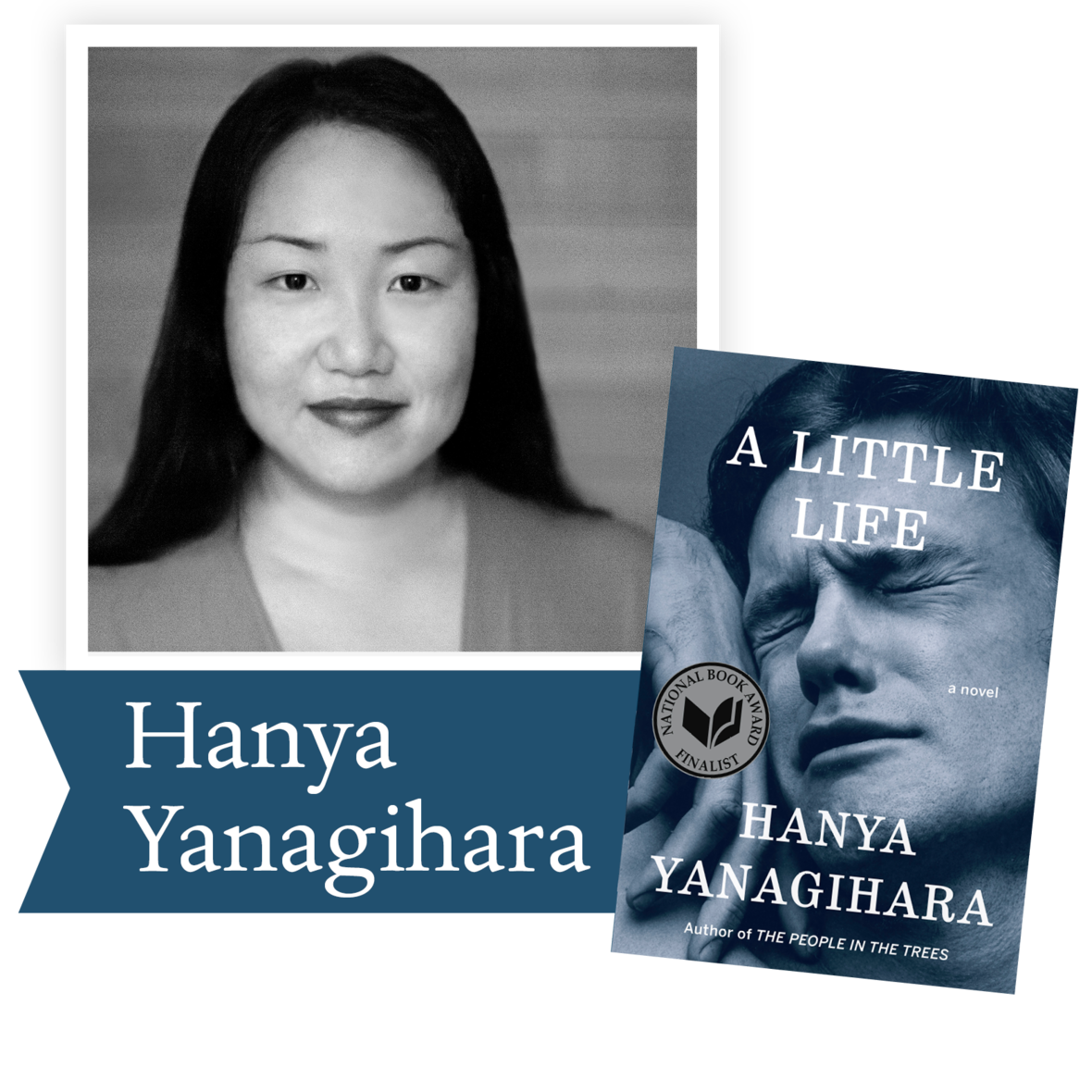A Little Life by Hanya Yanagihara (Doubleday): Four college classmates move to New York in search of fame and fortune. While their relationships, which are tinged by addiction, success, and pride, deepen over the decades, the men are held together by their devotion to the brilliant, enigmatic Jude, a man scarred by an unspeakable childhood trauma.

 table div table+table+table+table+table+table+table+table+table+table+table+table div table{width:100%;padding:0}table div table+table+table+table+table+table+table+table+table+table+table+table div table img{width:96.23%;padding:0;float:none}table div table+table+table+table+table+table+table+table+table+table+table+table div table td{width:100%;padding:0 1.88% 18px}/* styles */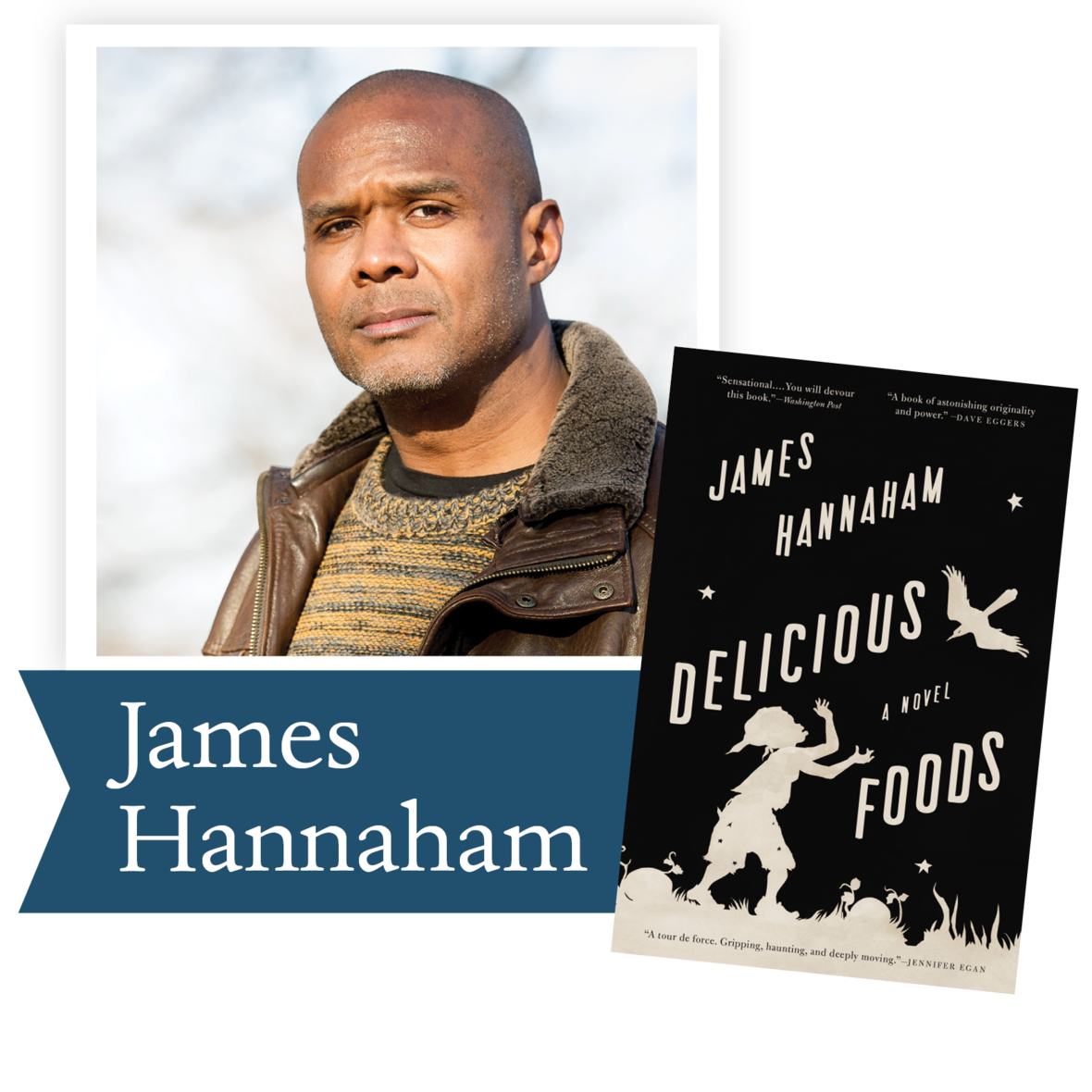Delicious Foods by James Hannaham (Little, Brown and Company): Held captive on a mysterious farm and under the sway of an overpowering addiction, a widow struggles to reunite with her young son in this uniquely American story of freedom, perseverance, and survival. The novel wrestles with timeless questions of love and freedom, forgiveness and redemption, tenacity and the will to survive.

 table div table+table+table+table+table+table+table+table+table+table+table+table+table+table div table{width:100%;padding:0}table div table+table+table+table+table+table+table+table+table+table+table+table+table+table div table img{width:96.23%;padding:0;float:none}table div table+table+table+table+table+table+table+table+table+table+table+table+table+table div table td{width:100%;padding:0 1.88% 18px}/* styles */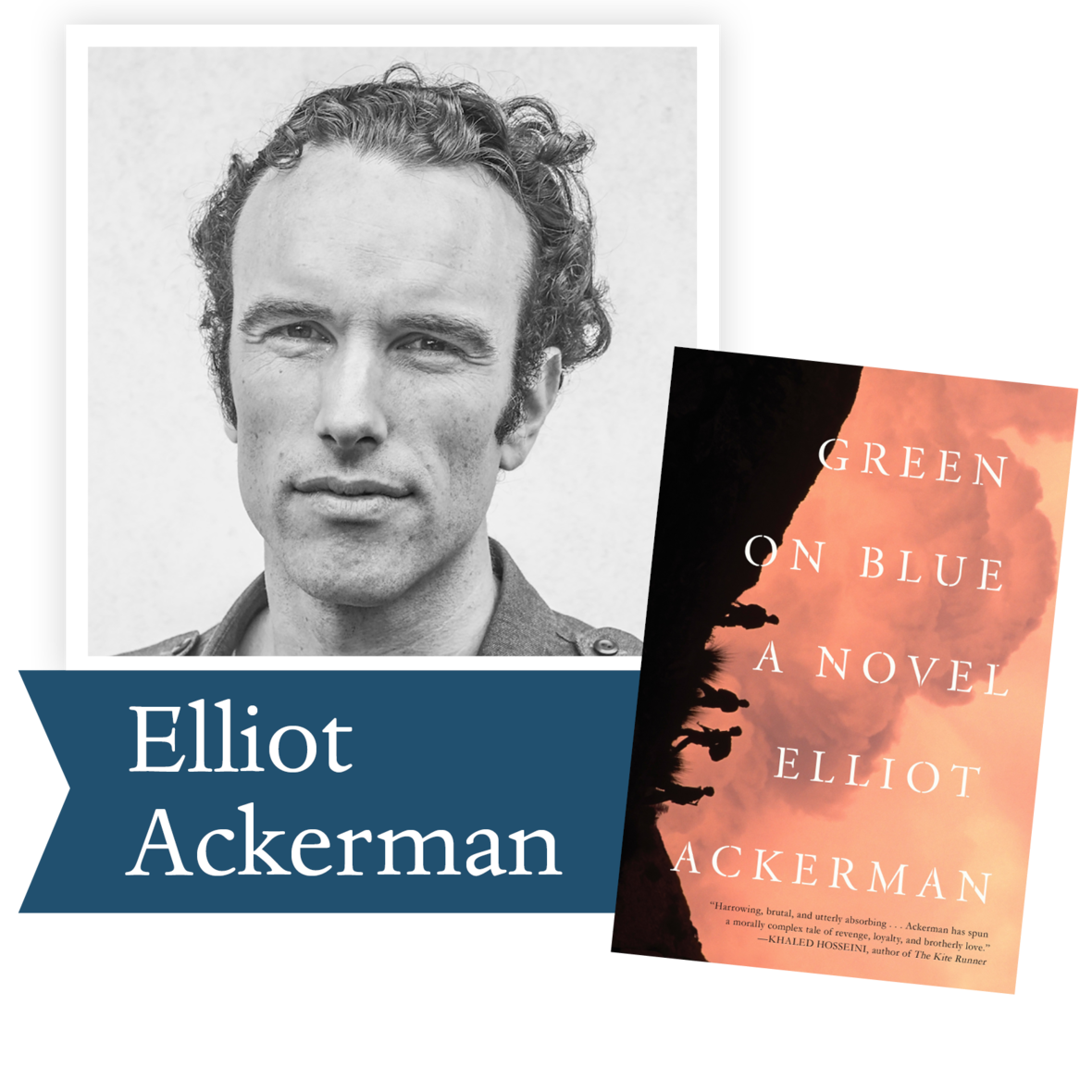Green on Blue by Elliot Ackerman (Scribner):
A young Afghan orphan is forced to join a US-funded militia to save his brother, who is hospitalized after an attack on their village. Written from the Afghan perspective, the novel is a gripping, morally complex story about boys caught in an elliptical war, and the sacrifices we make for love.

 table div table+table+table+table+table+table+table+table+table+table+table+table+table+table+table+table div table{width:100%;padding:0}table div table+table+table+table+table+table+table+table+table+table+table+table+table+table+table+table div table img{width:96.23%;padding:0;float:none}table div table+table+table+table+table+table+table+table+table+table+table+table+table+table+table+table div table td{width:100%;padding:0 1.88% 18px}/* styles */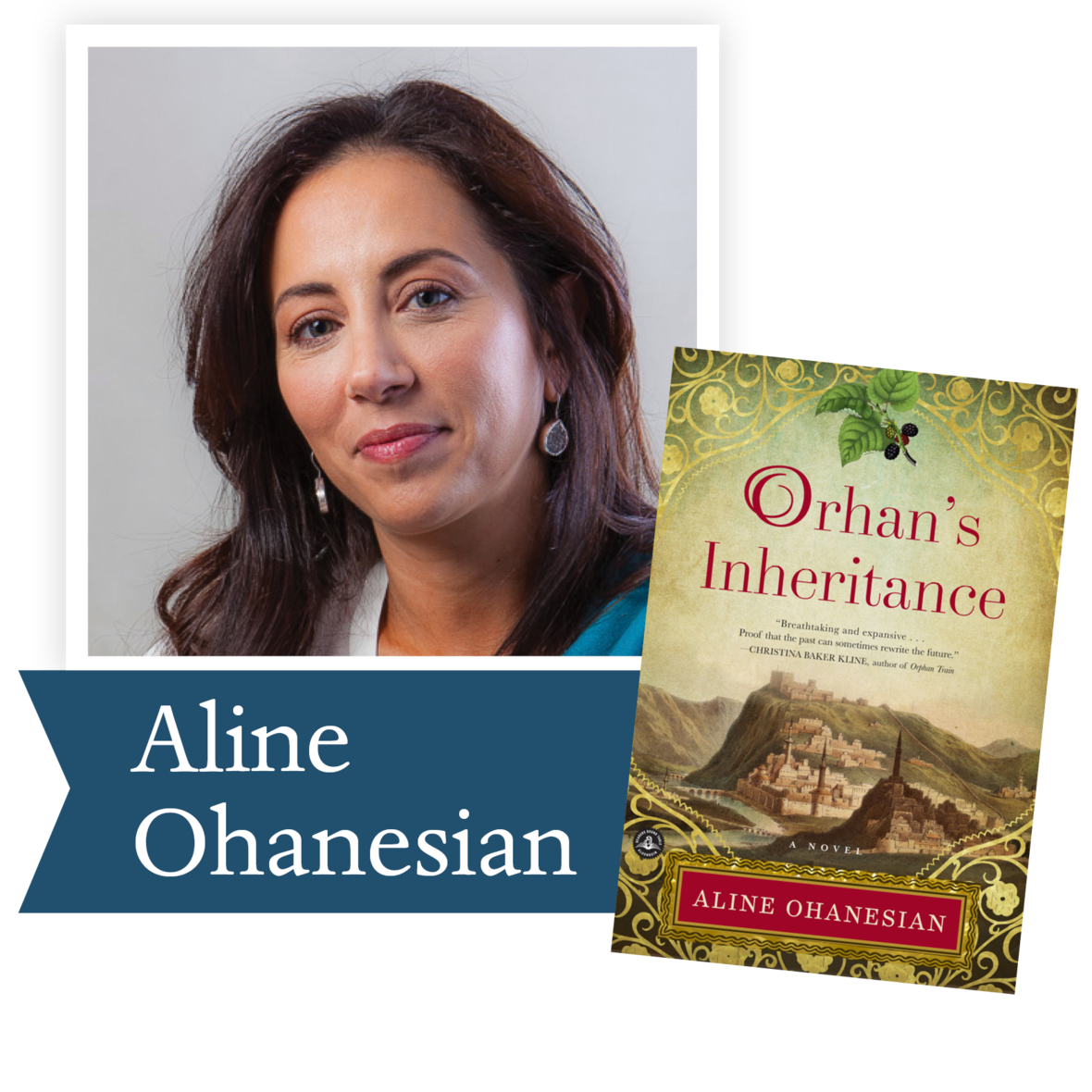Orhan’s Inheritance by Aline Ohanesian (Algonquin): When Orhan’s grandfather dies, Orhan inherits a decades-old kilim rug business. But the will raises more questions than it answers: his grandfather has left the family estate neither to his son Mustafa, nor to his grandson Orhan, but to a distant stranger, an aging woman in an Armenian retirement home in Los Angeles.

 table div table+table+table+table+table+table+table+table+table+table+table+table+table+table+table+table+table+table div table{width:100%;padding:0}table div table+table+table+table+table+table+table+table+table+table+table+table+table+table+table+table+table+table div table img{width:96.23%;padding:0;float:none}table div table+table+table+table+table+table+table+table+table+table+table+table+table+table+table+table+table+table div table td{width:100%;padding:0 1.88% 18px}/* styles */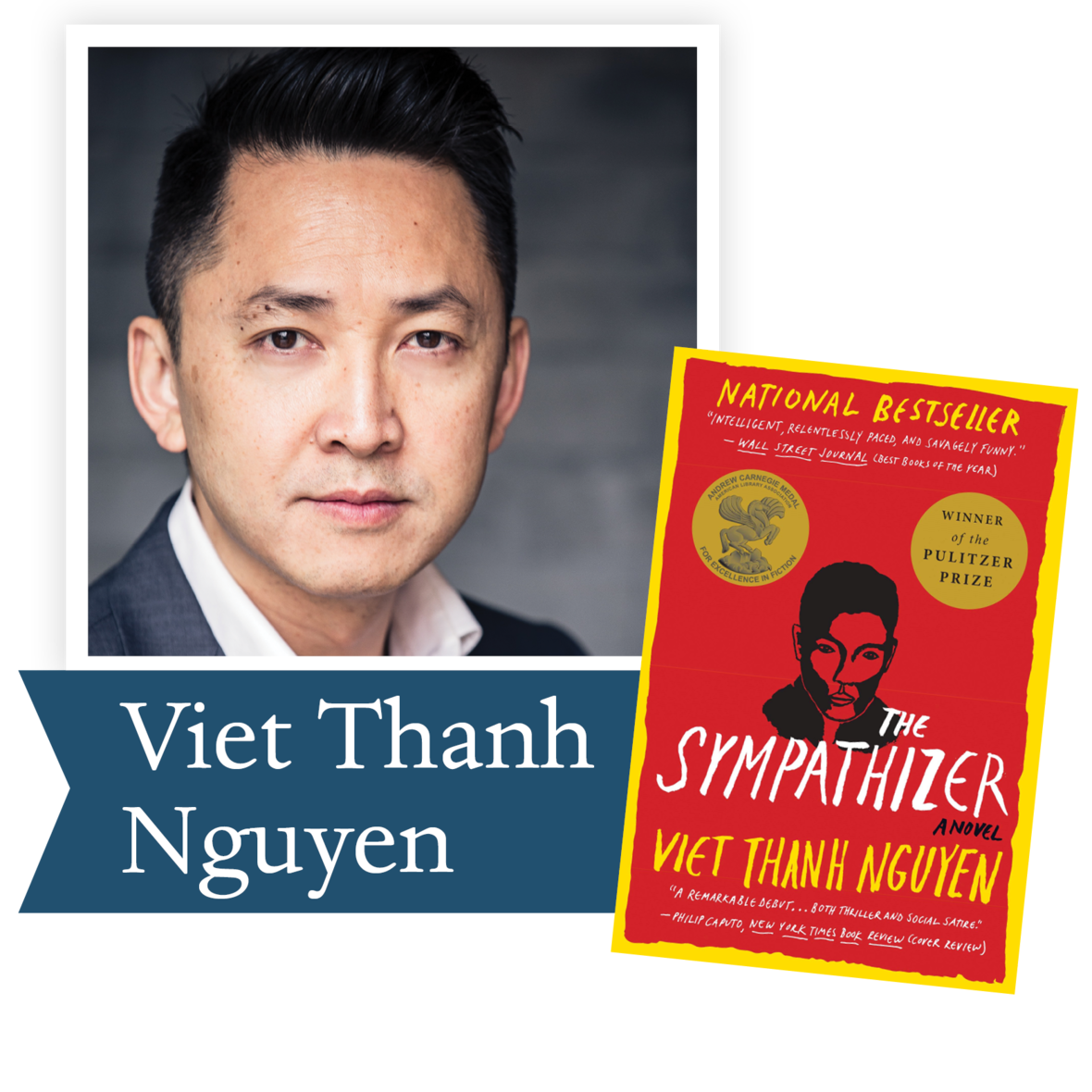The Sympathizer by Viet Thanh Nguyen (Grove Press): This is the story of a man of two minds, whose lofty ideals necessitate his betrayal of the people closest to him. A gripping spy novel, an astute exploration of extreme politics, and a moving love story, the novel explores a life between two worlds and examines the legacy of the Vietnam War.

 table div table+table+table+table+table+table+table+table+table+table+table+table+table+table+table+table+table+table+table+table div table{width:100%;padding:0}table div table+table+table+table+table+table+table+table+table+table+table+table+table+table+table+table+table+table+table+table div table img{width:96.23%;padding:0;float:none}table div table+table+table+table+table+table+table+table+table+table+table+table+table+table+table+table+table+table+table+table div table td{width:100%;padding:0 1.88% 18px}/* styles */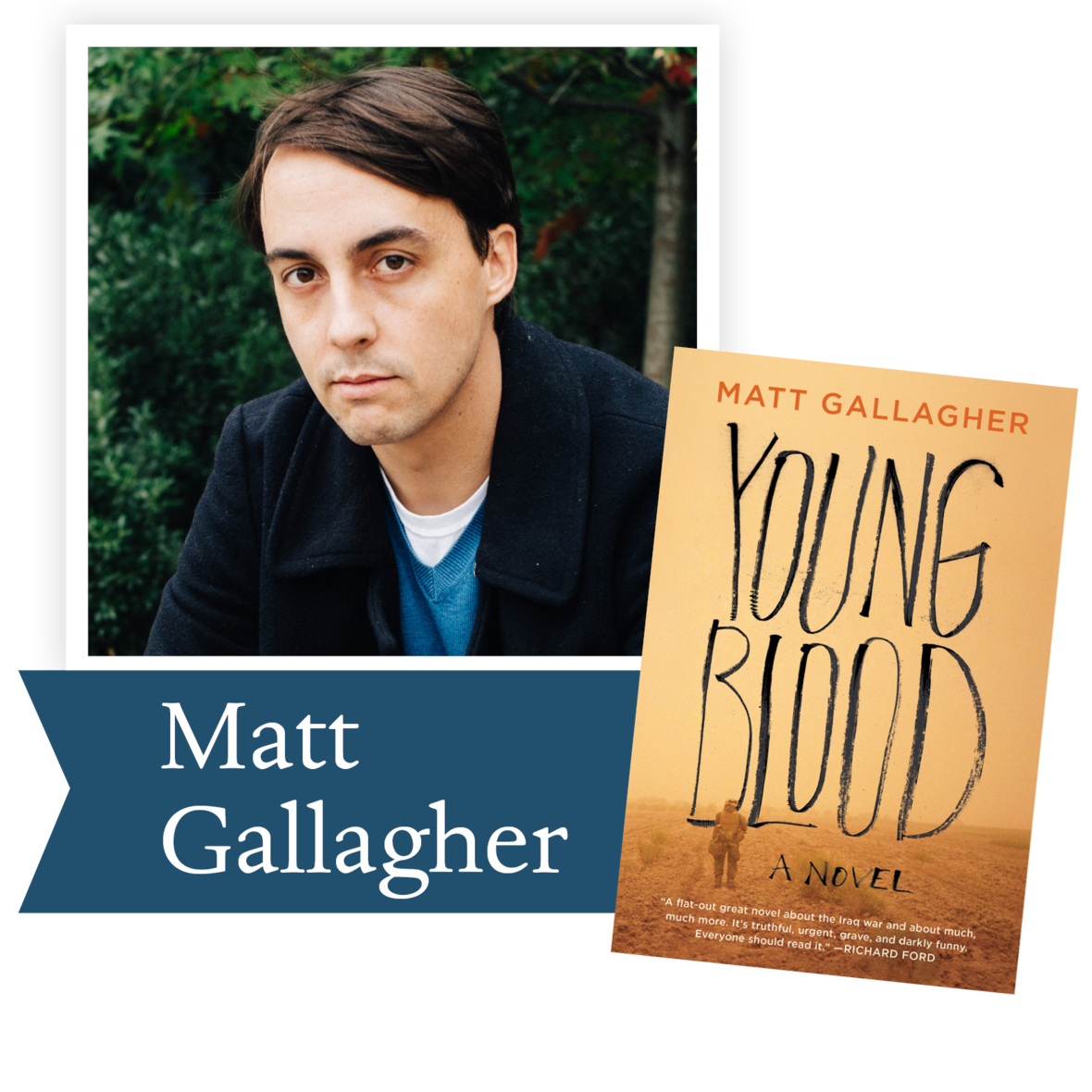Youngblood by Matt Gallagher (Atria): The US military is preparing to withdraw from Iraq, and newly minted lieutenant Jack Porter struggles to accept how it’s happening through alliances with warlords who have blood on their hands. He becomes obsessed with a tragic love story between a lost American soldier a local sheikh’s daughter, even as Iraq plunges back into chaos.

 table div table+table+table+table+table+table+table+table+table+table+table+table+table+table+table+table+table+table+table+table+table+table div table{width:100%;padding:0}table div table+table+table+table+table+table+table+table+table+table+table+table+table+table+table+table+table+table+table+table+table+table div table img{width:96.23%;padding:0;float:none}table div table+table+table+table+table+table+table+table+table+table+table+table+table+table+table+table+table+table+table+table+table+table div table td{width:100%;padding:0 1.88% 18px}/* styles */## 2016 Finalists - Nonfiction

 table div table+table+table+table+table+table+table+table+table+table+table+table+table+table+table+table+table+table+table+table+table+table+table+table div table{width:100%;padding:0}table div table+table+table+table+table+table+table+table+table+table+table+table+table+table+table+table+table+table+table+table+table+table+table+table div table img{width:96.23%;padding:0;float:none}table div table+table+table+table+table+table+table+table+table+table+table+table+table+table+table+table+table+table+table+table+table+table+table+table div table td{width:100%;padding:0 1.88% 18px}/* styles */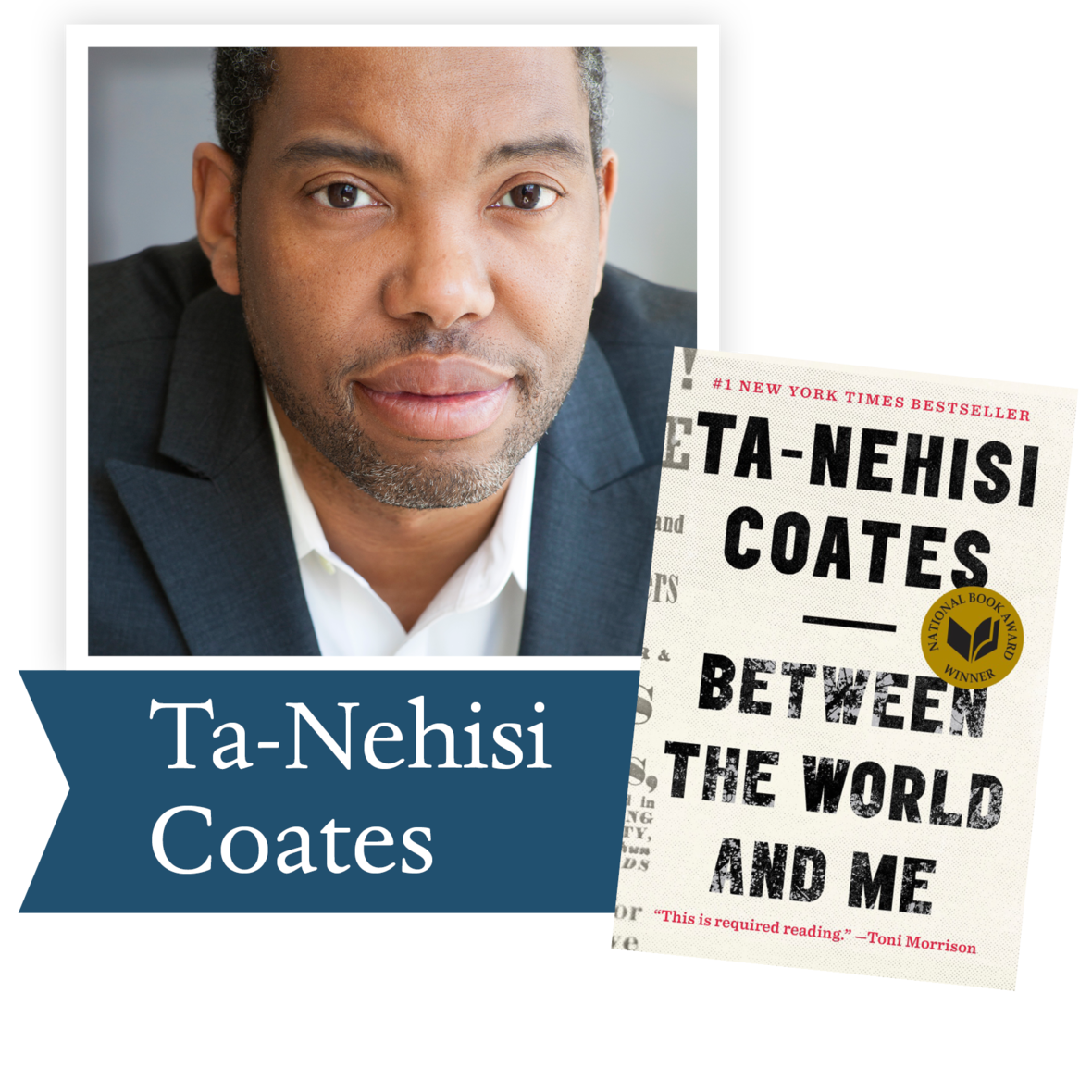Between the World and Me by Ta-Nehisi Coates (Spiegel & Grau): The author shares with his son—and readers—the story of his own awakening to the truth about history and his place in the world through a series of revelatory experiences. Taken together, these stories map a journey from fear and confusion to a full understanding of this country, this world and the pursuit of freedom.

 table div table+table+table+table+table+table+table+table+table+table+table+table+table+table+table+table+table+table+table+table+table+table+table+table+table+table div table{width:100%;padding:0}table div table+table+table+table+table+table+table+table+table+table+table+table+table+table+table+table+table+table+table+table+table+table+table+table+table+table div table img{width:96.23%;padding:0;float:none}table div table+table+table+table+table+table+table+table+table+table+table+table+table+table+table+table+table+table+table+table+table+table+table+table+table+table div table td{width:100%;padding:0 1.88% 18px}/* styles */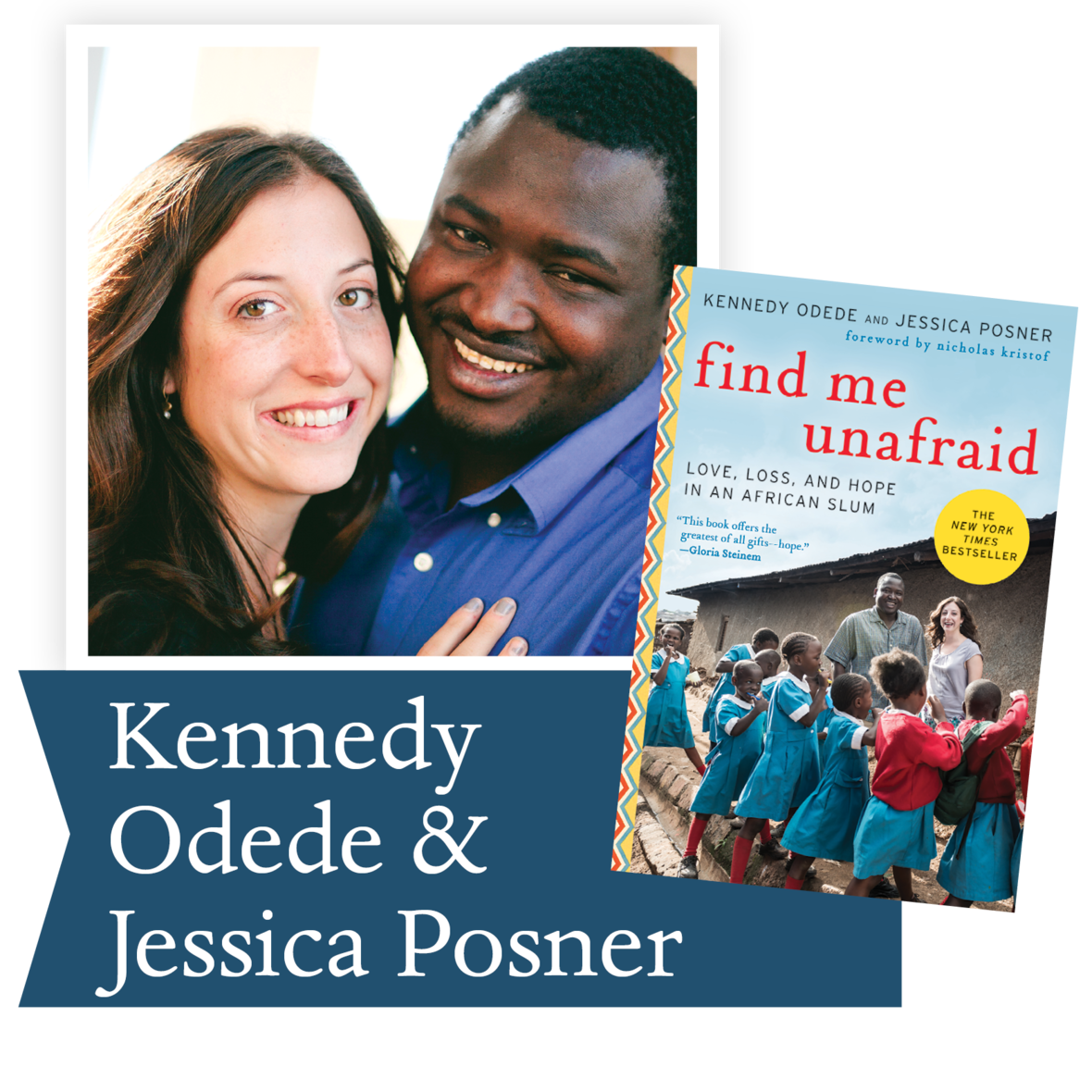Find Me Unafraid by Kennedy Odede and Jessica Posner (Harper Collins): This is the story of two young people from completely different worlds: he, from Kibera, Africa’s largest slum; and she, from Denver, Colorado. She comes to intern at the youth empowerment group he has started, and the two fall in love. Through their remarkable union, they are changing the lives of Kibera’s most vulnerable population: its girls.

 table div table+table+table+table+table+table+table+table+table+table+table+table+table+table+table+table+table+table+table+table+table+table+table+table+table+table+table+table div table{width:100%;padding:0}table div table+table+table+table+table+table+table+table+table+table+table+table+table+table+table+table+table+table+table+table+table+table+table+table+table+table+table+table div table img{width:96.23%;padding:0;float:none}table div table+table+table+table+table+table+table+table+table+table+table+table+table+table+table+table+table+table+table+table+table+table+table+table+table+table+table+table div table td{width:100%;padding:0 1.88% 18px}/* styles */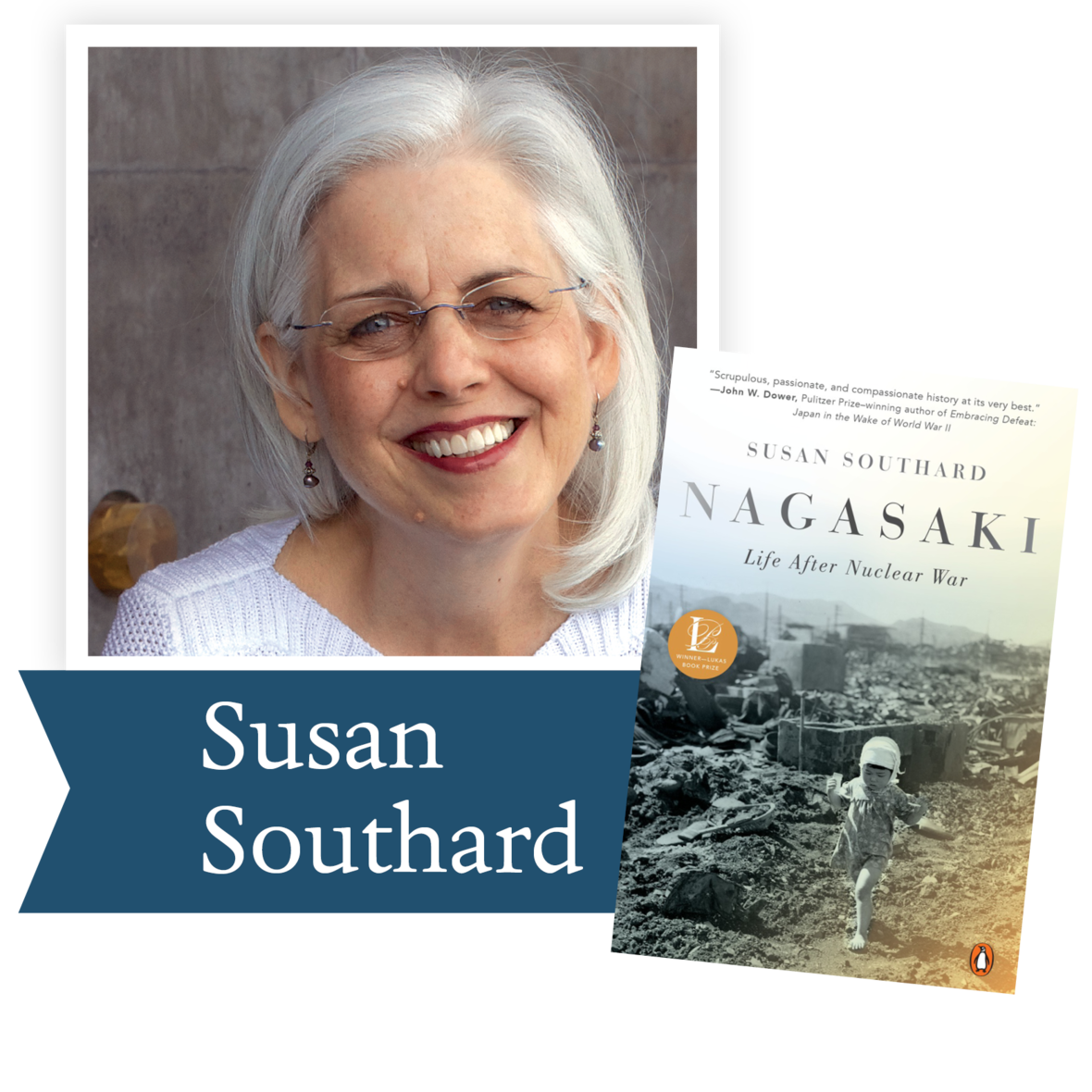Nagasaki by Susan Southard (Penguin Random House): The author relates the eyewitness accounts of five survivors of the bombing of Nagasaki – all teenagers at the time – intertwining their stories with examinations of their injuries and illnesses, as well as perceptions about the atomic bombings today. In this gripping narrative, a small number of survivors have spoken publicly to ensure history is never repeated.

 table div table+table+table+table+table+table+table+table+table+table+table+table+table+table+table+table+table+table+table+table+table+table+table+table+table+table+table+table+table+table div table{width:100%;padding:0}table div table+table+table+table+table+table+table+table+table+table+table+table+table+table+table+table+table+table+table+table+table+table+table+table+table+table+table+table+table+table div table img{width:96.23%;padding:0;float:none}table div table+table+table+table+table+table+table+table+table+table+table+table+table+table+table+table+table+table+table+table+table+table+table+table+table+table+table+table+table+table div table td{width:100%;padding:0 1.88% 18px}/* styles */Showdown by Wil Haygood (Knopf): The author details the life of Thurgood Marshall, the first African-American Supreme Court justice and one of the most transformative legal minds of the past one hundred years. Haygood creates a provocative look at Marshall’s life and the politicians, lawyers, activists, and others who shaped—or desperately tried to stop—the Civil Rights Movement.

 table div table+table+table+table+table+table+table+table+table+table+table+table+table+table+table+table+table+table+table+table+table+table+table+table+table+table+table+table+table+table+table+table div table{width:100%;padding:0}table div table+table+table+table+table+table+table+table+table+table+table+table+table+table+table+table+table+table+table+table+table+table+table+table+table+table+table+table+table+table+table+table div table img{width:96.23%;padding:0;float:none}table div table+table+table+table+table+table+table+table+table+table+table+table+table+table+table+table+table+table+table+table+table+table+table+table+table+table+table+table+table+table+table+table div table td{width:100%;padding:0 1.88% 18px}/* styles */The Reason You Walk by Wab Kinew (Penguin Random House): When his father was diagnosed with terminal cancer, Winnipeg broadcaster and musician Wab Kinew spent 2012 reconnecting with the accomplished but distant aboriginal man who'd raised him. Invoking hope, healing and forgiveness, The Reason You Walk is a poignant story of a towering but damaged father and his son as they embark on a journey to repair their family bond.

 table div table+table+table+table+table+table+table+table+table+table+table+table+table+table+table+table+table+table+table+table+table+table+table+table+table+table+table+table+table+table+table+table+table+table div table{width:100%;padding:0}table div table+table+table+table+table+table+table+table+table+table+table+table+table+table+table+table+table+table+table+table+table+table+table+table+table+table+table+table+table+table+table+table+table+table div table img{width:96.23%;padding:0;float:none}table div table+table+table+table+table+table+table+table+table+table+table+table+table+table+table+table+table+table+table+table+table+table+table+table+table+table+table+table+table+table+table+table+table+table div table td{width:100%;padding:0 1.88% 18px}/* styles */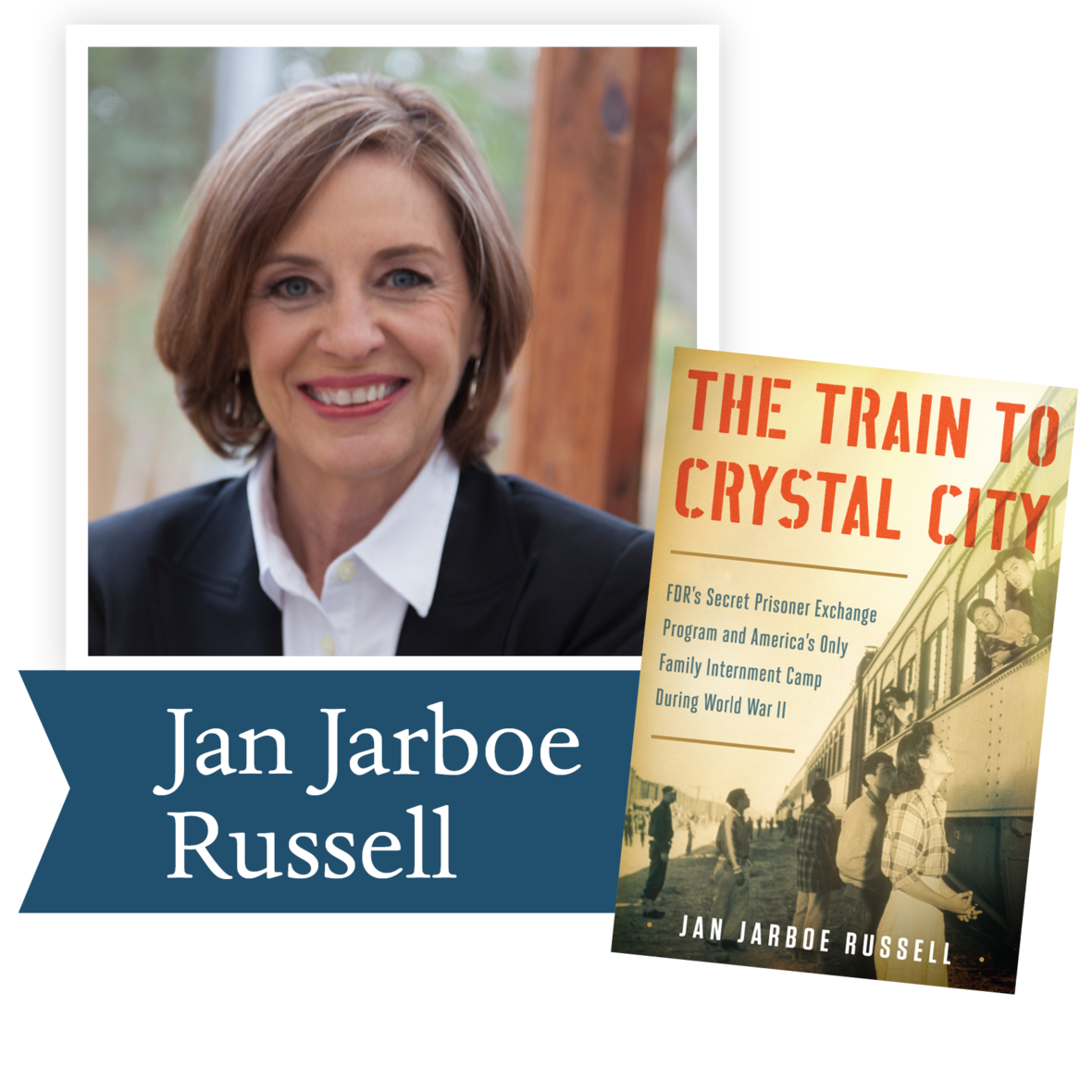The Train to Crystal City by Jan Jarboe Russell (Scribner): During World War II, thousands of families were incarcerated in a secret, FDR-approved American internment camp in Texas. The author reveals America’s war-time hysteria against the Japanese and Germans, FDR’s tactics to rescue high-profile POWs in Germany and Japan, and how the definition of American citizenship changed under the pressure of war.

 table div table+table+table+table+table+table+table+table+table+table+table+table+table+table+table+table+table+table+table+table+table+table+table+table+table+table+table+table+table+table+table+table+table+table+table+table div table{width:100%;padding:0}table div table+table+table+table+table+table+table+table+table+table+table+table+table+table+table+table+table+table+table+table+table+table+table+table+table+table+table+table+table+table+table+table+table+table+table+table div table img{width:96.23%;padding:0;float:none}table div table+table+table+table+table+table+table+table+table+table+table+table+table+table+table+table+table+table+table+table+table+table+table+table+table+table+table+table+table+table+table+table+table+table+table+table div table td{width:100%;padding:0 1.88% 18px}/* styles */## 2016 Ambassador Richard C. Holbrooke Distinguished Achievement Award Winner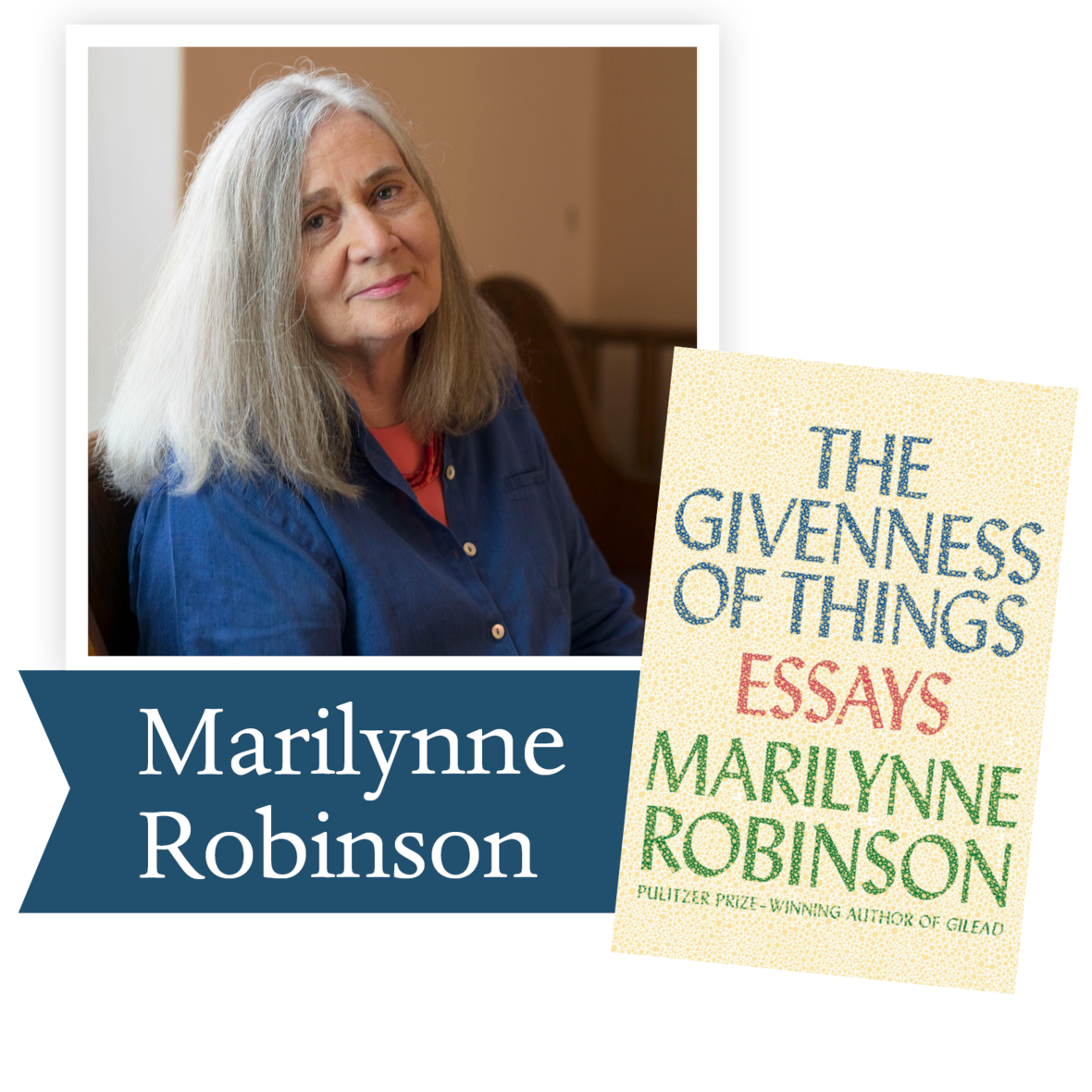As an introduction to the novels of Marilynne Robinson, we are quoting from the citation written by Carol Loranger, Associate Dean of the College of Liberal Arts at Wright State University.

“The novels, like most of the essays, have one-word titles—Housekeeping, Gilead, Home, and Lila—as though they too name the questions they will take up. But these questions have a more human scale than those of the essays and, as befits novels, pose the questions in terms of personal relationships. Her characters traverse the vast interpersonal spaces between belonging and alienation, most without ever leaving town. Each novel follows a small group of people, the last three centering on essentially the same community of people living in mid-twentieth-century middle-America, and the action is for the most part small: a prodigal returns; an elderly dying man contemplates his life and writes a letter to his son; a homeless young woman finds a home; a young woman leaves home and finds her true family. But the issues are big: the wages of America’s original sin of slavery; the care and keeping of the environment; the debt owed our children and their children; the burdens and blessings of reconciliation.”

The lives of the characters of Robinson’s novel are lived at a pace where they can be observed and absorbed, much in keeping with the lives of those who now spend time in quarantine—with time to read and to reflect.

If you'd like to read more about Marilynne's work, this great article is a compilation of The First Reviews of Every Marilynne Robinson Novel.

 table div table+table+table+table+table+table+table+table+table+table+table+table+table+table+table+table+table+table+table+table+table+table+table+table+table+table+table+table+table+table+table+table+table+table+table+table+table+table+table div table{width:100%;padding:0}table div table+table+table+table+table+table+table+table+table+table+table+table+table+table+table+table+table+table+table+table+table+table+table+table+table+table+table+table+table+table+table+table+table+table+table+table+table+table+table div table img{width:96.23%;padding:0;float:none}table div table+table+table+table+table+table+table+table+table+table+table+table+table+table+table+table+table+table+table+table+table+table+table+table+table+table+table+table+table+table+table+table+table+table+table+table+table+table+table div table td{width:100%;padding:0 1.88% 18px}/* styles */table div table+table+table+table+table+table+table+table+table+table+table+table+table+table+table+table+table+table+table+table+table+table+table+table+table+table+table+table+table+table+table+table+table+table+table+table+table+table+table+table+table div table{width:100%;padding:0}table div table+table+table+table+table+table+table+table+table+table+table+table+table+table+table+table+table+table+table+table+table+table+table+table+table+table+table+table+table+table+table+table+table+table+table+table+table+table+table+table+table div table img{width:96.23%;padding:0;float:none}table div table+table+table+table+table+table+table+table+table+table+table+table+table+table+table+table+table+table+table+table+table+table+table+table+table+table+table+table+table+table+table+table+table+table+table+table+table+table+table+table+table div table td{width:100%;padding:0 1.88% 18px}/* styles */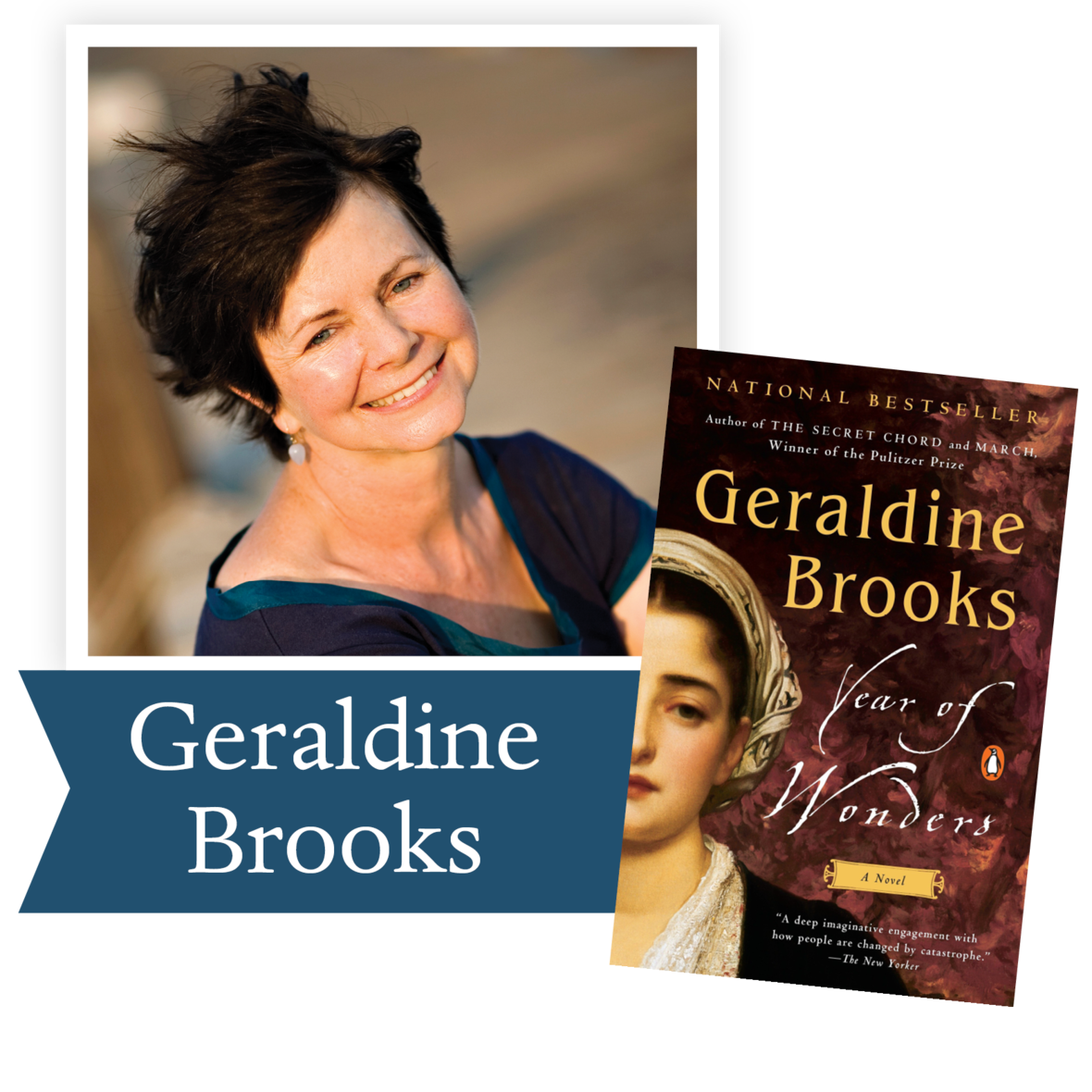“Plague stories remind us that we cannot manage without community . . . Year of Wonders is a testament to that very notion.” – The Washington Post

An unforgettable tale, set in 17th century England, of a village that quarantines itself to arrest the spread of the plague, from the author of The Secret Chord and of March, winner of the Pulitzer Prize.

Ron Rollins, Dayton Daily News Ideas and Voices Editor, will moderate the discussion of The Year of Wonders.

Email Emily Kretzer to be added to the list. We'll send details as they become available.

 table div table+table+table+table+table+table+table+table+table+table+table+table+table+table+table+table+table+table+table+table+table+table+table+table+table+table+table+table+table+table+table+table+table+table+table+table+table+table+table+table+table+table+table div table{width:100%;padding:0}table div table+table+table+table+table+table+table+table+table+table+table+table+table+table+table+table+table+table+table+table+table+table+table+table+table+table+table+table+table+table+table+table+table+table+table+table+table+table+table+table+table+table+table div table img{width:96.23%;padding:0;float:none}table div table+table+table+table+table+table+table+table+table+table+table+table+table+table+table+table+table+table+table+table+table+table+table+table+table+table+table+table+table+table+table+table+table+table+table+table+table+table+table+table+table+table+table div table td{width:100%;padding:0 1.88% 18px}/* styles */Support the Dayton Literary Peace Prize when you shop with Amazon using this link.